STEMLabs IQ
Lab 12 - M.A.D. Box

Now that you have explored what gears are and how they can be used to create a mechanical advantage, you will now calculate different gear ratios and combine them to obtain a compound gear ratio.

You will work in groups of four to calculate gear ratios and determine the resulting mechanical advantage.

### View an example

Begin by viewing the following example: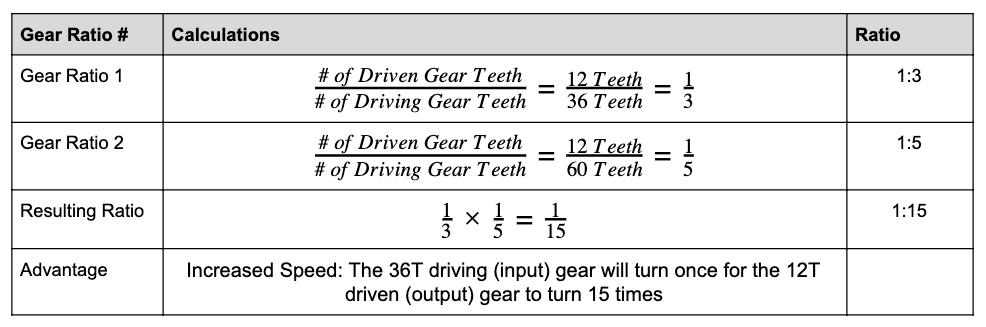In the example above, the Resulting Ratio row refers to calculating the Compound Gear Ratio by multiplying all of the individual gear ratios together.

Gear Ratio 1 has a 36 tooth-gear (36T gear) driving a 12 tooth-gear (12T gear). Viewing the relationship is Driven over Driving results in 12 over 36, which reduces down to one third. Thus, the ratio is 1:3.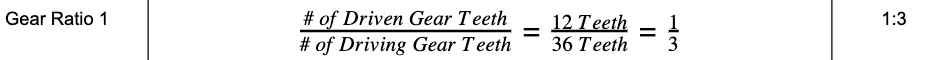Similarly for Gear Ratio 2, a 60T gear is driving a 12T gear. Viewing the relationship as Driven over Driving results in 12 over 60, which reduces to one fifth. Thus, the ratio is 1:5.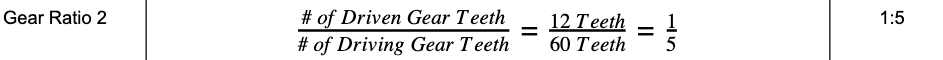To combine these two ratios, fraction multiplication is introduced. One third times one fifth is one fifteenth. Keep in mind, when multiplying fractions, you multiply straight across in the numerator and denominator. Thus, the compound gear ratio is 1:15.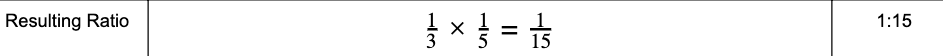Once the compounded gear ratio is calculated, it can now be determined what the mechanical advantage is. The resulting advantage is Increased Speed: The 36T driving (input) gear will turn once for the 12T driven (output) gear to turn 15 times.### Calculation 1

Fill in the missing calculations from the Gear Ratio table. Keep in mind, each person should be calculating according to their role.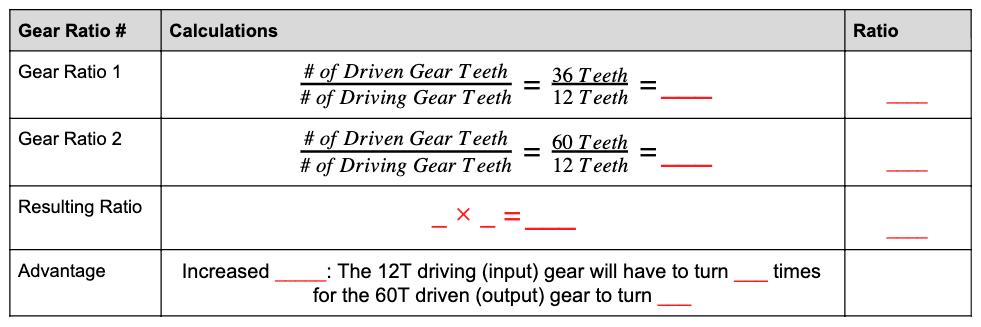• Role 1: Calculate the Gear Ratio 1 row of the above table. Show all work in your engineering notebook.

• Role 2: Calculate the Gear Ratio 2 row of the above table. Show all work in your engineering notebook.

• Role 3: Calculate the Resulting Ratio row of the above table. Check the calculations from Gear Ratio 1 and 2 before calculating the final compound gear ratio. Show all work in your engineering notebook.

• Role 4: Calculate the Advantage row of the above table. Show all work in your engineering notebook.

• All Roles: Once the table is completed, verify with all group members that the calculations are correct.